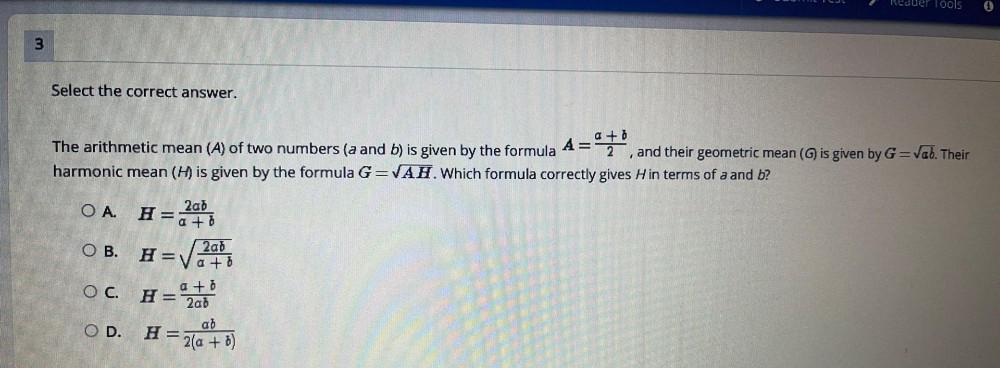Question:

# Reduer Tools 3 Select the correct answer. A= a + 0 2 The arithmetic mean (A) of two numbers (a and b) is given by the formula anReduer Tools 3 Select the correct answer. A= a + 0 2 The arithmetic mean (A) of two numbers (a and b) is given by the formula and their geometric mean() is given by G=Vab. Their harmonic mean (H) is given by the formula G=VAH. Which formula correctly gives Hin terms of a and b? OA. 2ab H= a +8 O B. Hrva O C. HE 2að a + a +0 2ab ab H= 2(a + b) - OD.